## Wednesday, July 18, 2007

### Irodov Problem 1.29

(a) time of flight can be found in any textbook explaining projectile motion and there is no need to explain it here.
(b) the range and the max height can also be found in any basic physics text book that explains projectile motion and I donot think I need to solve them here. For the angle at which maximum height is equal to range we have,(c) The equation of motion of the particle is given by,(d)I will provide two complete different ways to determining the radius of curvature, i) a purely mathematical approach and ii) a purely physics based approach.

The purely mathematics based approach: The raidus of curvature of a planar curve in Cartesian coordinates is given by,Here x' and y' are first derivatives and x'' and y'' are second derivatives. To find out how (1) came about please go here. I will not explain this since we focus on physics not math.

At time t = 0,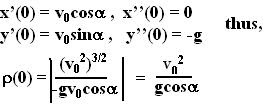Max height occurs at time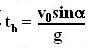. At this time,The purely physics based approach:
A more physics way to find the radius of curvature is to use the force diagram of the particle.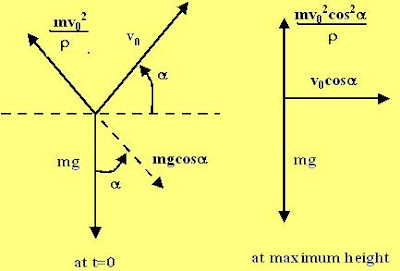Basically the idea is that at any point the moving particle experiences a centrifugal force (a pseudo force invented for convenience) that trying to push the particle in a direction perpendicular to its line of motion. This centrifugal force is to be balanced by gravity.

Initially the body moves at an angle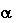to the horizontal at speed V0 and so the centrifugal force acts perpendicular to this and is equal to. Here, ofcourse the radius of curvature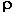of the particle is unknown and needs to be determined. The component of gravity that opposes the centrifugal force, as shown in the figure, is given by,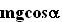. Since we know that the particle is not acceleration along this direction,Using the same explanation as above for the case when the particle is at its maximum height we have,This is exactly what we obtained using the purely math approach!!! The use of pseudo force definitely makes things very easy dosen't it!!